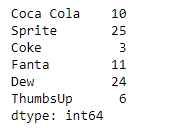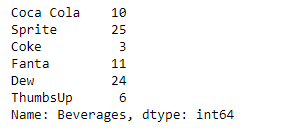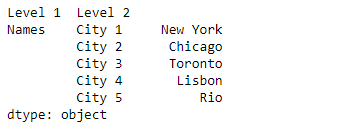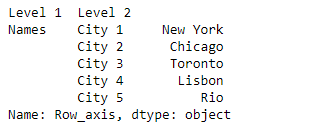# Python | Pandas Series.rename()

• Last Updated : 14 Oct, 2019

Pandas series is a One-dimensional ndarray with axis labels. The labels need not be unique but must be a hashable type. The object supports both integer- and label-based indexing and provides a host of methods for performing operations involving the index.

Pandas` Series.rename()` function is used to alter Series index labels or name for the given Series object.

Syntax: Series.rename(index=None, **kwargs)

Parameter :
index : dict-like or functions are transformations to apply to the index
copy : Also copy underlying data
inplace : Whether to return a new Series. If True then value of copy is ignored.
level : In case of a MultiIndex, only rename labels in the specified level.

Returns : Series, DataFrame, or None

Example #1: Use `Series.rename()` function to rename the name of the given Series object.

 `# importing pandas as pd``import` `pandas as pd`` ` `# Creating the Series``sr ``=` `pd.Series([``10``, ``25``, ``3``, ``11``, ``24``, ``6``])`` ` `# Create the Index``index_ ``=` `[``'Coca Cola'``, ``'Sprite'``, ``'Coke'``, ``'Fanta'``, ``'Dew'``, ``'ThumbsUp'``]`` ` `# set the index``sr.index ``=` `index_`` ` `# Print the series``print``(sr)`

Output :Now we will use `Series.rename()` function to rename the name of the given series object.

 `# rename the series``result ``=` `sr.rename(``'Beverages'``)`` ` `# Print the result``print``(result)`

Output :As we can see in the output, the `Series.rename()` function has successfully renamed the given series object.

Example #2: Use `Series.rename()` function to rename the MultiIndex axis of the given Series object.

 `# importing pandas as pd``import` `pandas as pd`` ` `# Creating the Series``sr ``=` `pd.Series([``'New York'``, ``'Chicago'``, ``'Toronto'``, ``'Lisbon'``, ``'Rio'``])`` ` `# Create the MultiIndex``index_ ``=` `pd.MultiIndex.from_product([[``'Names'``], [``'City 1'``, ``'City 2'``, ``'City 3'``, ``'City 4'``, ``'City 5'``]],``                                                                      ``names ``=``[``'Level 1'``, ``'Level 2'``])`` ` `# set the index``sr.index ``=` `index_`` ` `# Print the series``print``(sr)`

Output :Now we will use `Series.rename()` function to rename the 0th level of the given series object.

 `# rename the 0th level``result ``=` `sr.rename(level ``=` `0``, index ``=` `'Row_axis'``)`` ` `# Print the result``print``(result)`

Output :As we can see in the output, the `Series.rename()` function has successfully renamed the 0th level of the given series object.

My Personal Notes arrow_drop_up